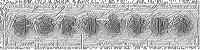What Ifs:  Gravitons

Gravitons are particles with high gravitational fields and minimal if not zero volume. These particles can then be used to modify gravitational forces between objects.

High concentrations of gravitons in any locality would cause an increase in the gravitational force towards that locality.

Before anything can be done we must find gravitons, after that is accomplished we will then be able to attempt to alter the movement of gravitons independent from the source of gravitons. The simplest way could be to find things gravitons are attracted to, likely large quantities of mass, or other gravitons. It would be helpful to have anti-gravitons which has the opposite effect of gravity, it repels mass, and other gravitons. In this framework we find that anti-gravitons are attracted to anti-gravitons, and gravitons are attracted to gravitons, and not each other. This runs contradictory to our current day same repels, different attracts paradigm. Maybe that is why we have not discovered these particles, since it appears that electricity and magnetism are both discovered and we know what can generate these forces, they both have properties that like particles repel and different particles attract.

Technologies such as sheilding from gravitons should be researched. If there is a material or field in which the gravitational effects of gravitons are void, we can in effect transport large quantities of gravity anywhere. We would be able to store these gravitons until it is needed to be used. If there is a material or field in which gravitons can not flow through, yet the gravitational forces are still felt on the other side of this barrier then this material is useful when we need the gravitational force to flow in a certain direction. We also need to find a medium in which gravitons flows freely with minimal impedence(a gravity conductor in a sense). Once we have these things, we can create graviton flow, and graviton store.

What would happen if we coiled the conductor around a bar, and established a flow through the coil?

What would happen if we coiled another wire onto the previous bar at different turn ratios?

What would happen if we applied a flow in the second wire while there is a flow in the first?
In the opposite direction?

What would happen if the coil was a torroid?

Can we use this to alter our momentum? We first direct a large quantity of gravitons to be released at high velocities towards the direction we wish to change our momentum, the gravitons are volumeless but that does not affect momentum, they might be massless in which case the gravitons would pull us in the direction we release them in, while we suffer no mass recoil. In the case that gravitons contain mass, we would need to know the mass to gravity ratio of gravitons, if mass is greater than gravity, this method would not gain us much use, but if mass is less than gravity, we can still potentially release it in the direction and still change our momentum although not as much.